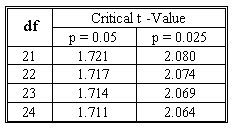### CFA Practice Question

There are 208 practice questions for this study session.

### CFA Practice Question

Beth has two independent variables and 25 observations in her regression analysis. The t-statistic for one of the independent variables X1 is 1.98. What is the critical value for Student's t distribution? Is the variable significant at the 5% confidence level? Assume 2-tailed test.Correct Answer: The critical value is 2.074, and the variable is not significant.

The degrees of freedom: 25 - 2 - 1 = 22.
t0.025, 22: 2.074.
As 1.98 < 2.074, the variable is not significant at the 5% confidence level.